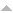## Publications

Export 5 results:
Sort by: Author [ Title] Type Year
N
Fernandesh, V. U. "A new class of divisors of semigroups of isotone mappings of finite chains." Izv. Vyssh. Uchebn. Zaved. Mat. (2002): 51-59.
Fernandes, Vitor H. "Normally ordered inverse semigroups." Semigroup Forum. 56 (1998): 418-433.Website
Fernandes, Vítor H. "Normally ordered semigroups." Glasg. Math. J.. 50 (2008): 325-333.Website
Fernandes, Vítor H., and Teresa M. Quinteiro. "A note on bilateral semidirect product decompositions of some monoids of order-preserving partial permutations." Bull. Korean Math. Soc.. 53.2 (2016): 495-506. AbstractWebsite

In this note we consider the monoid $PODI_n$ of all monotone partial permutations on $\{1,\ldots,n\}$ and its submonoids $DP_n$, $POI_n$ and $ODP_n$ of all partial isometries, of all order-preserving partial permutations and of all order-preserving partial isometries, respectively. We prove that both the monoids $POI_n$ and $ODP_n$ are quotients of bilateral semidirect products of two of their remarkable submonoids, namely of extensive and of co-extensive transformations. Moreover, we show that $PODI_n$ is a quotient of a semidirect product of $POI_n$ and the group $\mathcal{C}_2$ of order two and, analogously, $DP_n$ is a quotient of a semidirect product of $ODP_n$ and $\mathcal{C}_2$.

Dimitrova, I., Vítor H. Fernandes, and J. Koppitz. "A note on generators of the endomorphism semigroup of an infinite countable chain." Journal of Algebra and its Applications (DOI: 10.1142/S0219498817500311). 16 (2017): 1750031 (9 pages). AbstractWebsite

In this note, we consider the semigroup $O(X)$ of all order endomorphisms of an infinite chain $X$ and the subset $J$ of $O(X)$ of all transformations $\alpha$ such that $|Im(\alpha)|=|X|$. For an infinite countable chain $X$, we give a necessary and sufficient condition on $X$ for $O(X) = < J >$ to hold. We also present a sufficient condition on $X$ for $O(X) = < J >$ to hold, for an arbitrary infinite chain $X$.